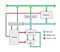# Example Programs for Calcutron-33

This is a follow up story on my story about inventing a RISC CPU called Calcutron-33 which has a decimal based number system rather than a binary number system.

Obviously this has no practical application for actual implementation. Rather the intention here is to come up with a simple model of a CPU, which is easy to teach.A diagram of our simple Decimal RISC CPU, showing how data flows to different parts. It is the Decoder which decides what parts are enabled and thus where data can flow in. Decode only knows what to enable by looking at Instruction Register, which contains latest instruction fetched from memory.

The idea here is to play with some example programs which can be useful for a beginner to implement. What I am interested in figuring out, is what a minimal set of features are to be able to do something interesting. Such as how many instructions do we need to support? How many registers do we need at minimum?

Get two inputs, add them together and write them to output

`loop:    INP x1    INP x2    ADD x1, x2    OUT x1    BRA loop`

## Doubling Inputs

Repeatedly double inputs and write to output.

`loop:    INP x1    ADD x1, x1    OUT x1    BRA loop`

## Reverse Inputs

Take two inputs and reverse their order

`loop:    INP x1    INP x2    OUT x2    OUT x1    BRA loop`

## Multiply by 8

Multiply each input by 8. This is the first step to understand how addition can be used for multiplication.

`loop:    INP x1    ADD x1, x1    ADD x1, x1    ADD x1, x1    OUT x1    BRA loop`

## Multiply by 3

Sometimes you need multiple registers to achieve multipliation

`loop:    INP x2    ADD x1, x2, x2    ADD x1, x2    OUT x1    BRA loop`

## Zero Exterminator

Remove all the zeros. Early way to learn the use of branching

`loop:    INP x1    BRZ x1, loop    OUT x1    BRA loop`

## Maximizer

Pick the largest of two numbers

`loop:    INP x1    INP x2    SUB x1, x1, x2    BGT x1, firstsecond:    OUT x2    BRA loop    first:    OUT x1    BRA loop`

## Simple Multiplier

The most primitive form of general multiplier. It picks two numbers at a time, multiplies them and outputs result.

`loop:    INP x1    INP x2    CLR x3    multiply:    ADD x3, x1    DEC x2    BGT x2, multiply    OUT x3        BRA loop`

## Fast Multiplier

The is program from original story, for how to multiple numbers.

Unlike the simple multiplier above we use a lot fewer iterations by using shifts. This speeds up the multiplication.

`    INP x2  // first number to multiply. This will get added    INP x3  // second number. Treated as counter    CLR x1  // accumulator for result. Clear it out.    nextdigit:    RSH x4, x3, 1  // Push right most digit of x3 into x4    multiply:    ADD x1, x1, x2 // Add first input to accumulator         DEC x4         // Decrement counter for number of additions    BGT x4, multiply  // Repeat while x4 > 0        LSH x2, x2, 1     // Left shift. x2 made 10x larger    BGT x3, nextdigit // check if all digits have been processed        OUT x1`

# Implementation of Calcutron-33

This made up CPU instruction set architecture now has a Julia package, Calcutron33.jl offering an assembler, disassembler and simulator.

It is still in early stages, but several of these example programs should work.

Written by